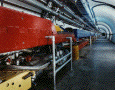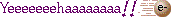Accelerators Accelerators solve two problems for physicists. First, since all particles behave like waves, physicists use accelerators to increase a particle's momentum, thus decreasing its wavelength enough that physicists can use it to poke inside atoms. Second, the energy of speedy particles is used to create the massive particles that physicists want to study.How do accelerators work? Basically, an accelerator takes a particle, speeds it up using electromagnetic fields, and bashes the particle into a target or other particles. Surrounding the collision point are detectors that record the many pieces of the event. Question: What is the nearest particle accelerator to you right now? [ Answer ] How do physicists get the particles they want to study?